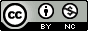## Monday, September 21, 2009

### Comparison of plots using Stata, R base, R lattice, and R ggplot2, Part I: Histograms

One of the nicer things about many statistics packages is the extremely granular control you get over your graphical output.  But I lack the patience to set dozens of command line flags in R, and I'd rather not power the computer by pumping the mouse trying to set all the clicky-box options in Stata's graphics editor.  I want something that just looks nice, using the out-of-the-box defaults.  Here's a little comparison of 4 different graphing systems (three using R, and one using Stata) and their default output for plotting a histogram of a continuous variable split over three levels of a categorical variable.

First I'll start with the three graphing systems in R: base, lattice, and ggplot2.  If you don't have the last two packages installed, go ahead and download them:

install.packages("ggplot2")
install.packages("lattice")

Now load these two packages, and download this fake dataset I made up containing 100 samples each from three different genotypes ("geno") and a continuous outcome ("trait")

library(ggplot2)
library(lattice)

Now let's get started...

R: base graphics

par(mfrow=c(3,1))
with(subset(mydat,geno=="aa"),hist(trait))
with(subset(mydat,geno=="Aa"),hist(trait))
with(subset(mydat,geno=="AA"),hist(trait))

R: lattice

histogram(~trait | factor(geno), data=mydat, layout=c(1,3))

R: ggplot2

qplot(trait,data=mydat,facets=geno~.)

# Update Tuesday, September 22, 2009
# A commenter mentioned that this code did not work.
# If the above code does not work, try explicitly
# stating that you want a histogram:
qplot(trait,geom="histogram",data=mydat,facets=geno~.)

Stata

insheet using "http://people.vanderbilt.edu/~stephen.turner/ggd/2009-09-21-histodemo.csv", comma clear
histogram trait, by(geno, col(1))

Commentary

In my opinion ggplot2 is the clear winner.  Again I'll concede that all of the above graphing systems give you an incredible amount of control of every aspect of the graph, but I'm only looking for what gives me the best out-of-the-box default plot using the shortest command possible. R's base graphics give you a rather spartan plot, with very wide bins.  It also requires 4 lines of code.  (If you can shorten this, please comment).  By default, the base graphics system gives you counts (frequency) on the vertical axis.  The lattice package in R does a little better perhaps, but the default color scheme is visually less than stellar.  Also, I'm not sure why the axis labels switch sides every other plot, and the ticks on top of the plot are probably unnecessary.  I still think the bins are too wide.  You lose some information especially on the bottom plot towards the right tail.  The vertical axis is proportion of total.  Stata's default plot looks very similar to lattice, but again uses a very unattractive color scheme.  It uses density for the vertical axis, which may not mean much to non-statisticians.  The default plot made by ggplot2 is just hands-down good-looking.  There are no unnecessary lines delimiting the bins, and the binwidth is appropriate.  The vertical axis represents counts.  The black bars on the light-gray background have a good data-ink ratio.  And it required the 2nd shortest command, only 3 characters longer than the Stata equivalent.

I'm ordering the ggplot2 book (Amazon, ~\$50), so as I figure out how to do more with ggplot2 I'll post more comparisons like this.  If you use SPSS, SAS, MATLAB, or something else, post the code in a comment here and send me a picture or link to the plot and I'll post it here.

1.Your ggplot code does not work for me.

"Error: ggplot: Some aesthetics (y) are missing sceles..."

Don't you forgot to set "geom" parameter?

I am new to ggplot. I am looking forward to more examples. Honestly, this one does not convince me that ggplot2 is worth learning.

2.Hmm... worked for me just now. Are you using the most up to date version of ggplot2? I'm not sure how new the qplot function is, but that's what I'm using here.

qplot apparently knew I wanted a histogram based on how I called it, but you could explicitly state that you want a histogram:

qplot(trait,geom="histogram",data=mydat,facets=geno~.)

Alternatively you could use the longwinded ggplot function, but I don't know yet how to use this syntax and get the three plots to line up vertically.

ggplot(mydat, aes(trait)) + geom_histogram() + facet_wrap(~geno)

Learning R has a much more extensive comparison of lattice and ggplot2 including commentary from ggplot2's creator in a 14 part series here.

3.I am sorry. You are right - I did not use the most recent version.

I have installed ggplot2 yesterday. However, I was using R 2.6.2 and therefore I got version 0.6. The current version is 0.8.3.

4.Great post. I agree that for basic exploratory analysis, its important to have a graphic system that is efficient. When it comes to a finished product for inclusion in the report, then programming control and lots of options are more important to me.

The following are two ways to produce plots in base graphics with fewer lines of code.

par(mfrow=c(3,1))
sapply(airquality[1:3], hist)

par(mfrow=c(3,1))
for(i in seq(3)) hist(airquality[[i]], main = names(airquality)[i], xlab = names(airquality)[i])

The first is concise but does not have pretty labels.
the second is a little longer but does have labels.

5.You can do this in two lines as well with the base package :

For example :

val <- c(rnorm(500),rpois(500,6),rnorm(500,sd=2))
cod <- c(rep('a',500),rep('b',500),rep('c',500))

par(mfcol=c(1,3))
lapply(split(val,cod),hist,col='grey',frequency=TRUE,ylim=c(0,200),xlim=range(val))

6.In regards to your ggplot2 example for multiple histograms, do you know if there is an
a)easy way to reorder the facets OR
b)relabel the facet components?

For example, if you wanted from top to bottom the labels to read 'Aa','aa','AA' (and correspond with their respective graphs).

The facet command seems to order the information alphabetically. I read through the files you posted on July 27, 2010 from Hadley Wickham's short course (paired with multiple google searches) but haven't been able to figure out how to reorder or relabel a properly ordered set of histograms.

Thanks.